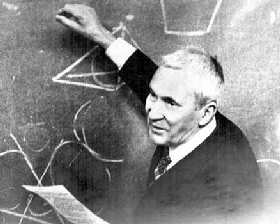A# Kolmogorov, Andrei Nikolaievich (1903–1987)Andrei Kolmogorov was a Russian mathematician and physicist who advanced the foundations of probability theory, and the algorithmic theory of randomness and made crucial contributions to the foundations of statistical mechanics, stochastic processes, information theory, fluid mechanics, and nonlinear dynamics. All of these areas, and their interrelationships, underlie complex systems, as they are studied today. His work on reformulating probability started with a 1933 paper in which he built up probability theory in a rigorous way from fundamental axioms, similar to Euclid's treatment of geometry. Kolmogorov went on to study the motion of the planets and turbulent fluid flows, later publishing two papers in 1941 on turbulence that even today are of fundamental importance.

In 1954 he developed his work on dynamical systems in relation to planetary motion, thus demonstrating the vital role of probability theory in physics and reopening the study of apparent randomness in deterministic systems, much along the lines originally conceived by Henri Poincaré. In 1965 he introduced the algorithmic theory of randomness via a measure of complexity, now referred to as Kolmogorov complexity. According to Kolmogorov, the complexity of an object is the length of the shortest computer program that can reproduce the object. Random objects, in his view, were their own shortest description. Whereas, periodic sequences have low Kolmogorov complexity, given by the length of the smallest repeating "template" sequence they contain. Kolmogorov's notion of complexity is a measure of randomness, one that is closely related to Claude Shannon's entropy rate of an information source.